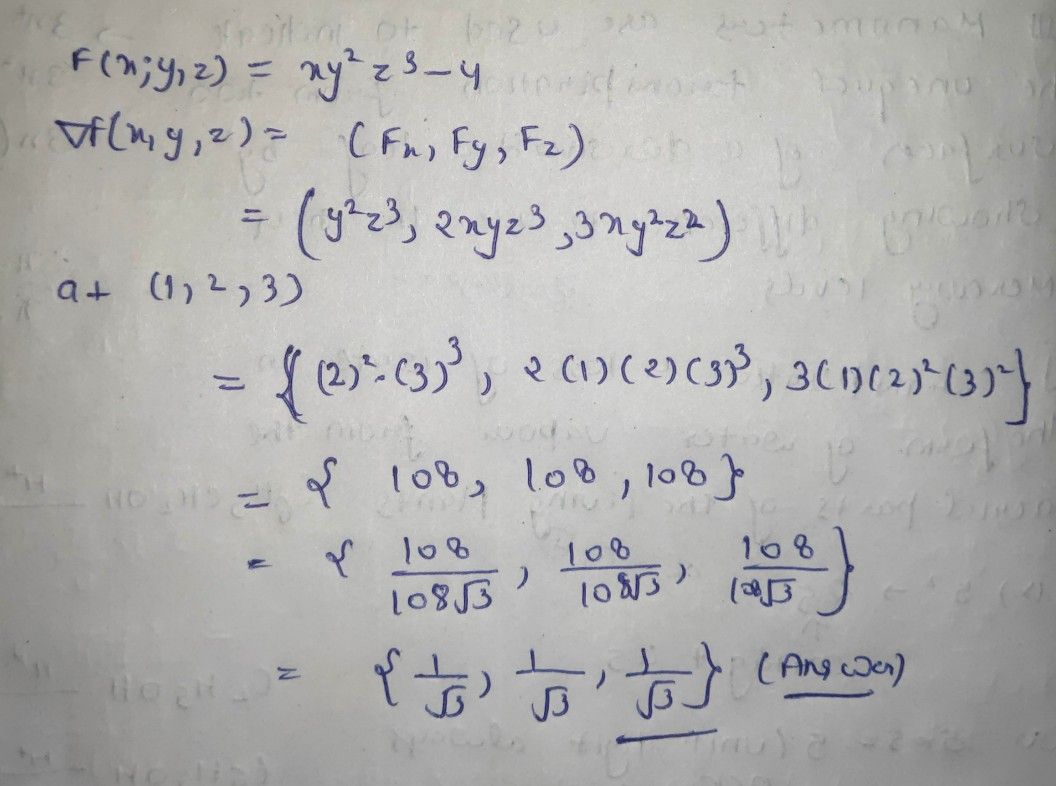Symbol
Problem$\left(a\right)$ Find the unit vector normal to the surface $xy^{2}z^{3}=4$ $at$ the point $\left(1,2,3\right).$
Other
Search count: 111
SolutionQanda teacher - AskhinaThanks for asking a question. You can ask any doubt without any hesitation in a direct way also for high-quality answers quickly and please rate 5 stars if you are satisfied with my answers And, if you have no doubt related to the previous question then please evaluate the answer and give some coins as a gift.Student
thanks a ton mam
one more questionQanda teacher - Askhina
yes
yes but dear plz understand to me only one question is allowed to give in one time (one chat room) by QandA...
u can ask again by preffered match
I think you may understand to me and qanda policies